Reach Us+32-10-28-02-25
Solutions of the Ultra-Relativistic Euler Equations in Riemann Invariants | OMICS International
Journal of Physical Mathematics
All submissions of the EM system will be redirected to Online Manuscript Submission System. Authors are requested to submit articles directly to Online Manuscript Submission System of respective journal.

# Solutions of the Ultra-Relativistic Euler Equations in Riemann Invariants

Abdelrahman MAE* and Moaaz O

Department of Mathematics, Faculty of Science, Mansoura University, Egypt

*Corresponding Author:
Abdelrahman MAE
Professor, Department of Mathematics
Faculty of Science, Mansoura University, Egypt
Tel: +20502242388
E-mail: [email protected]

Received Date: March 06, 2017; Accepted Date: April 25, 2017; Published Date: April 28, 2017

Citation: Abdelrahman MAE, Moaaz O (2017) Solutions of the Ultra-Relativistic Euler Equations in Riemann Invariants. J Phys Math 8: 222. doi: 10.4172/2090-0902.1000222

Copyright: © 2017 Abdelrahman MAE, et al. This is an open-access article distributed under the terms of the Creative Commons Attribution License, which permits unrestricted use, distribution, and reproduction in any medium, provided the original author and source are credited.

Visit for more related articles at Journal of Physical Mathematics

#### Abstract

In this paper we introduce a new technique for constructing solutions of the ultra-relativistic Euler equations. The Riemann invariants are formulated. We also give some applications of the Riemann invariants. We firstly study the geometric properties of the solution in Riemann invariants coordinates. The other application of Riemann invariants, representing the ultra-relativistic Euler equations in diagonal form, which admits the existence of global smooth solution for the ultra-relativistic Euler equations.

#### Keywords

Relativistic Euler system; Hyperbolic systems; Shock waves; Entropy conditions; Rarefaction waves; Riemann invariants; Diagonal form

#### Introduction

This paper is devoted to the analysis of the following coupled ultrarelativistic Euler system: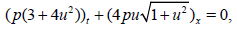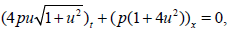(1)

Where p>0 and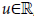, [1-8].

Consider x=x(t) is a shock-discontinuity of the weak solution of (1) with speed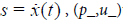the state lower to the shock and (p+, u+) the state upper to the shock with p± >0, respectively. Then the Rankine-Hugoniot jump (RHj) conditions are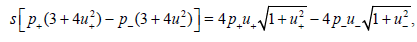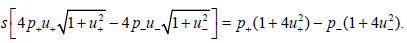(2)

The entropy inequality at singular points is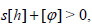(3)

where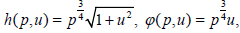which is equivalent to u_ >u+, .

We can rewrite the 2×2 system for p and u in (1) in the quasilinear form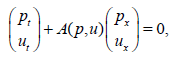(4)

where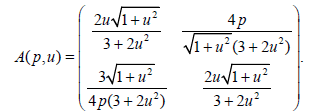The eigenvalues of that system (1) are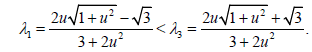(5)

The characteristic velocities λ1 and λ3 are corresponding to the 1 and 3 family of waves, respectively. The decoupled equation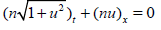(6)

for the particle density n > 0 gives rise for contact discontinuities with the eigenvalue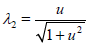, 

Lemma 1.1 Suppose that (p_,u_)=(p_,0) and (p+, u+)= (p, u(p)) satisfy condition (2). Then the shock curves satisfy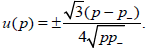(7)

The +ve sign in (7) with p < p_ gives a 3-shock. These 3-shocks satisfy both the RHj conditions (2) and the entropy condition (3), or in a similar way u_> u+.

The -ve sign in (7) with p_ < p gives a 1-shock. These 1-shocks satisfy both the RHj conditions (2) and the entropy condition (3), or in a similar way u_> u+ . Furthermore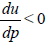on shock curves S1 and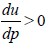on shock waves S3, where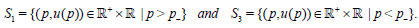(8)

we studied the Riemann invariants for the ultra-relativistic Euler system. In fact we show that the Riemann invariants have interesting relations with the representations of nonlinear elementary waves (shocks and rarefaction waves). Namely, we points out the relation between Riemann invariants and nonlinear elementary waves. This turns out to be the basic ingredient of our paper . One of the main applications of the Riemann invariants is to derive the diagonal form of system (1). We hope that these formula will be useful in various studies of the ultra-relativistic Euler system, for example, in developing numerical methods, . We show that the Riemann invariants, play a pivotal role in the solution of the ultra-relativistic Euler system (1).

In other words, in this paper we pose the following questions:

1. What are the Riemann invariants.

2. For what purpose they are useful.

The rest of this paper is given as follows : In Section 2, we derive the Riemann invariants for the ultra-relativistic Euler equations (1). In fact this topic plays a useful role in studying the ultra-relativistic Euler system (1) in a completely unified way. In Section 3 we give some applications of the Riemann invariants for the system (1). The first one, we study the geometric properties of the solution in the Riemann invariants coordinates. we give a new parametrization of the system (1), namely Lemma 3.1. This parametrization plays an important role in order to study these properties in useful way. The second one, is to give the diagonal form of the ultra-relativistic Euler system (1). Finally, in Section 4 we give the conclusions.

#### Riemann Invariants

We derive the Riemann invariants for the system (1), which plays a main role in this paper.

We consider our rarefaction waves. If we assume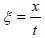, then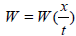satisfies the ordinary differential equation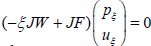where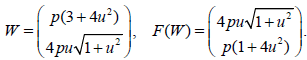(9)

and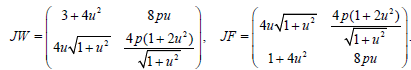(10)

If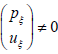, then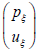is an eigenvector of JW-1JF for the eigenvalue ξ. Since JW-1 JF has two distinct real eigenvalues, λ13, there are two families of rarefaction waves, 1-rarefaction waves and 3-rarefaction waves.

We first consider 1-rarefaction waves. The eigenvector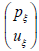satisfies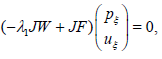(11)

From (10) we get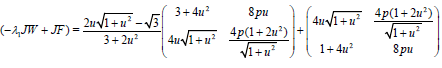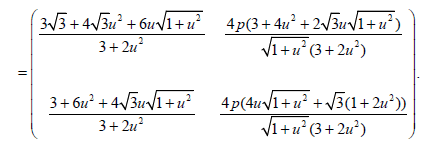(12)

Using (11), we have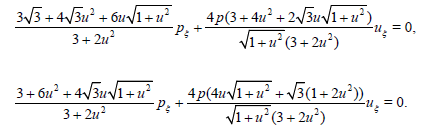(13)

The two equations are dependent since

Det(−λ1 JW + JF) = 0.

So we have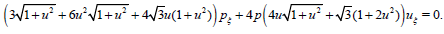Thus we obtain the following differential equation,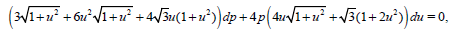which has the solution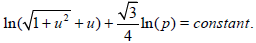This is the 1-rarefaction curve. Similarly, we can determine the 3-rarefaction as follows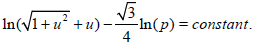Since Riemann invariants are functions, which are constant along rarefaction waves, we can define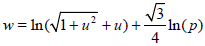(14)

and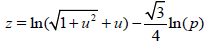(15)

are the 1 and 3-Riemann invariant for system (1), respectively.

Remark 2.1 The function w=w (p,u) is constant across 1-rarefaction waves and z=z (p,u) is constant across 3-rarefaction waves.

Lemma 2.1 The mapping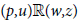is one-to-one with nonsingular Jacobian for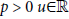. 

#### Applications of Riemann Invariants

In this section we will show how Riemann invariants can be used to solve various problems related to the system (1).

Geometry of the shock waves

Here we study the geometry of the shock waves of the ultrarelativistic Euler system (1) in the Riemann invariants coordinates (w,z). We first derive the new parametrization of the ultra-relativistic Euler system (1) in Lemma 3.1. In fact this representation turns out to be very valuable in order to study the geometric properties of the solution in a unified way.

Lemma 3.1 Assume that (p_,u_) and (p+, u+) ≡ (p, u) satisfy the jump condition (2). Then the following relations hold: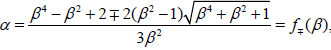(16)

where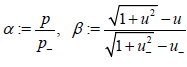. The -ve sign in (16) and p _< p gives a 1-shock curve S1 given in (8). The +ve sign in (16) and p < p_ gives a 3-shock curve S3 given in (8).

Proof. Using the RHj conditions (2) and eliminating the shock speed S give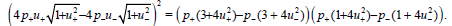Now multiplying out gives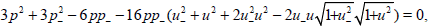that is,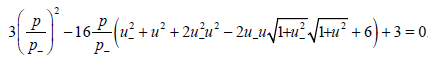After a straight but tedious computation, we get the result explained in Figure 1

The following lemma shows that the differences Z - Z_ and w - w_ through a shock curve depend only on the parameters α, and thus the geometric aspect of the shock wave in the zw-plane is independent of the base point. To give this lemma in a useful way we define the functions,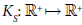by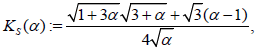(17)

and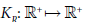by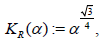(18)

for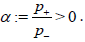Lemma 3.2 Let z=z(p+, u+) w=w(p+, u+). Then the representation of 1-shock curve S1 for the system (1) based at (z_w_) with respect to the parameter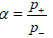is given as follows: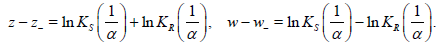While the 3-shock curves S3 based at (z_w_) has the parametrization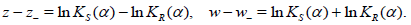Lemma 3.3 The 3-shock wave based at an arbitrary point (w_, z_) is the reflection in the wz-plane of the 1-shock wave based at the same point, where the axis of reflection is the line passing through (w_, z_), parallel to the line w=z.

Proof. Using (16), then the result follows immediately from the following: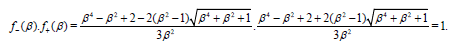The following lemma presents further important features of the shock wave.

Lemma 3.4 For shock curves of system (1) we have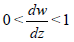(19)

along a 1-shock curves S1 and(20)

a along a 3-shock curves S3.

Therefore we can use either the pu-plane or the zw-plane to study our model, see Figure 1. Thus we conclude that the shock waves are independent of the base point (z_w_).

Diagonalization of the ultra-relativistic Euler equations

Here we present the ultra-relativistic Euler system (1) in diagonalized form. This form enables us to develop numerical methods to study the ultra-relativistic Euler system (1), . This will be presented in a forthcoming paper.

Definition 3.1 System (4) is said to be diagonalizable, if there exists a smooth transformationR=(w,z)T with non-vanishing Jacobian such that (4) can be rewritten as follows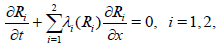(21)

Where λi(Ri) are smooth function of Riemann invariants R.

The diagonal system (21) is so important possessing so interesting properties. For example, it is easier to find exact solutions and study uniqueness of solutions, [11,12]. In fact, not all quasilinear systems can represent in diagonal form. Hence, it is so interesting to study this problem.

Proposition 3.1 The diagonalized system for system (1) is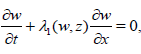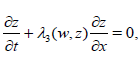(22)

where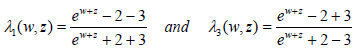illustrated in Figure 2.

Proof. We first start with the first equation of (22), namely with λ1(w,z) One can follows with λ3(w,z) similarly. From (5), we get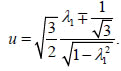Hence we have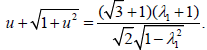(23)

Another equivalent form of the same relation, using (14) and (15) is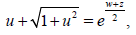(24)

From (23) and (24) and after a straight but tedious computation, we obtain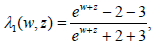hence the proof of the proposition is completed.

Remark 3.1 In fact the diagonal formula is very useful in developing numerical methods, see .

Remark 3.2 Based on the results given in, Theorem 2.4, Lemma 3.1], in order to prove the existence of the global smooth solution on t ≥ 0 for system (1), it is sufïcient to prove that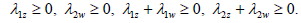One can easily check the following: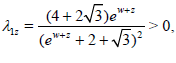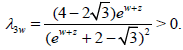We also have λ1w1z and λ3w3z hence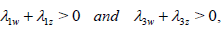which completes the statement.

#### Conclusions

In this work, we presented the Riemann invariants method for the ultra-relativistic Euler equations. We have shown that the shock curves have good geometry in Riemann invariant coordinates. The diagonal form of the ultra-relativistic Euler system has been introduced, which admits the existence of global smooth solution.

#### References

Select your language of interest to view the total content in your interested language

### Article Usage

• Total views: 1517
• [From(publication date):
June-2017 - Nov 14, 2019]
• Breakdown by view type
• HTML page views : 1378Can't read the image? click here to refresh